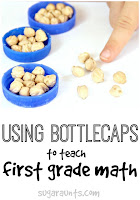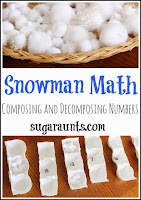Base Tens Operations with Paper Clips | The OT Toolbox

## Base Tens Operations with Paper Clips

In first grade math, base ten is a big part of learning the basics of adding.  Kids need to understand that the two digits of a two-digit number represent amounts of tens and ones.  This is a basis of more complex math problems.  So what is a base ten?  Base 10 refers to the position of a number.  There is a ones place value, a tens place value, and a one hundreds place value in a three digit number like 236.  Each number is 10 times the value to the right of it.  A ten is thought of as a bundle of ones.  Using base ten concepts, kids can decipher that a two digit number is made up of one or more tens and single numbers.  We made these base ten manipulatives using paper clips for comparing, adding, and counting.  The bonus to this activity is that it is a fine motor workout!## Base Ten Activities for Kids:

This post contains affiliate links.
We used the paper clipsto first, count out ten.  We attached the ten paper clips to create a tens value.  We then built more and counted by ten.  Using the strand of tens paper clips, we were able to build 7 strands of tens. (Then we ran out of paper clips!)

• Using strands of tens, sort them into piles.  You might have three strands of tens in one pile and 4 strands of tens in the other pile.  Ask your child to figure out which is more.
• Compose numbers using strands of the ten paper clips and ones.  Write down numbers (You can even use our homemade worksheets to write out numbers.)  Then help your child build the numbers using the strands of tens and the individual paper clips.
• Subtract multiples of 10 using the paper clips. Write out the problems and discuss the methods and reasoning.
• Add multiples of 10 using the paper clips.  Write out the problems and discus methods and reasoning.
• Tell your child a two digit number and ask them to mentally find 10 more or 10 less than the number.  Use the paper clips to figure out the answer if needed.
• Add within 100, including adding a two-digit number and a one-digit number, using the paper clips as a model.  Draw out pictures of the problem.  Write out the problem.
• Use the paper clips to add two-digit numbers, adding strands together and ones together.
• Discuss how to use the paperclips to compose a ten.  See how we recently practiced composing numbers.
How many ways can you think of to use paper clips in math with base ten activities?

Try more of our math activities:We are posting this idea as part of our month-long Learning with Free Materials series where we provide 31 days of learning using free or almost free materials in homeschooling or school-based extension learning like homework.  This post is part of the 31 Days of Homeschooling Tips as we blog along with other bloggers with learning at home tips and tools.